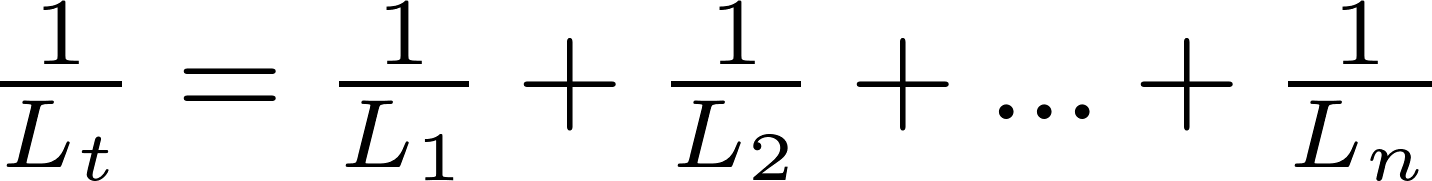# Inductors in parallel

Inductors can be connected in parallel to decrease to total inductance. The total inductance is equal to the reciprocal (inverse) of the sum of the reciprocal of all individual inductances.

### Formula